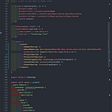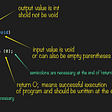# Write Your Own RSA Encryption Tool — Part 1 starting with theory

RSA, the most famous name in cryptography, what is it? In this series, I will walk you through the all you need to know about RSA — from the mathematical theory behind it to the final implementation in Python.

First, we have to learn one fundamental operation: mod, or modular arithmetic. What is mod, well it is simple, take a look at the following true statements, and you will get an idea of what mod is doing:

5 (mod 3) = 2, 4 (mod 3) = 1, 3 (mod 3) = 0, 7 (mod 3) = 1…

Well, do you recognize something? Hope you do! Mod operation is really looking for the reminder of the number on the outside of the parentheses divided by the number inside the parentheses. Or, we say

a (mod b) = c if a = b * n + c for some integer n.

Some more “complex” example might be like 23432 (mod 23 = 18), 1323 (mod 84) = 63 and ect. Sometimes, we also write as: a = (c mod b).

Then, we move forward with it. Can we do addition in modular arithmetic system? In other words, if we have

a = (c mod b) and d = (e mod b), do we have something like (a+d) = (c+e mod b)? The answer is Yes. Not surprisingly, similar rules should hold for subtraction, multiplication as well. In fact, below is list of properties hold for modular operation from wikipedia page:

However, you might notice that I deliberately left something out: division. In fact division might not work in the modular system. Take a look at the following example:

Consider in the mod 6 settings:

we have 2 = (2 mod 6). Then 2*2 = (4 mod 6), but we also have 2*5 = (4 mod 6). Then, what will be the result of 4/2 mod (6), will that be 2 or 5? This causes confusion as we see (one operation leading to multiple potential answer), so division is not always well defined here.

Another interesting difference between modular operation is that we do have multiplicative inverse, meaning a * a^(-1) = 1, which we do not have for integers. (2 * 1/2 = 1, but 1/2 is not an integer). One simple example is mod — 3 system: 2 * 2 = (1 mod 3). So 2 is itself’s multiplicative inverse. However, not all numbers in all the modular system have multiplicative inverse. The importance of multiplicative inverse will be shown in future articles.

Now, enough about theory part. It’s time to write some code. The code is extremely simple. For any two numbers a and b, you can just compute a mod b by directly typing `a % b` in Python. Feel free to play around with it a little bit.

Some nice challenges are left to you:

1. Use Python to compute the modular system of 3,5,7,13,15. Is every number in those systems have multiplicative inverse?
2. Can you try to solve the equation x² = (a mod b) in any modular system? If it is not always possible, can you try to come up with an example. (Search the keywords Quadratic Residue )

Happy learning & coding :)

--

--

--

## More from Tech & Math

SDE at FAANG. Love to talk about tech and math

Love podcasts or audiobooks? Learn on the go with our new app.

## Why I don’t use useEffect to set data in Redux## How To Create a Bot That Tweets Quotes## Taking a closer look at the Network Stack## Azure Functions — Bindings and triggers explained by an example using C### File Management for a Creative in 2019## Running PNETLab on GCP## Tech & Math

SDE at FAANG. Love to talk about tech and math

## How to write your first function in C — main(); printf();## Five Irrefutable Reasons Why Kids Should Learn to Code## Why is Python the best choice for Android app development?